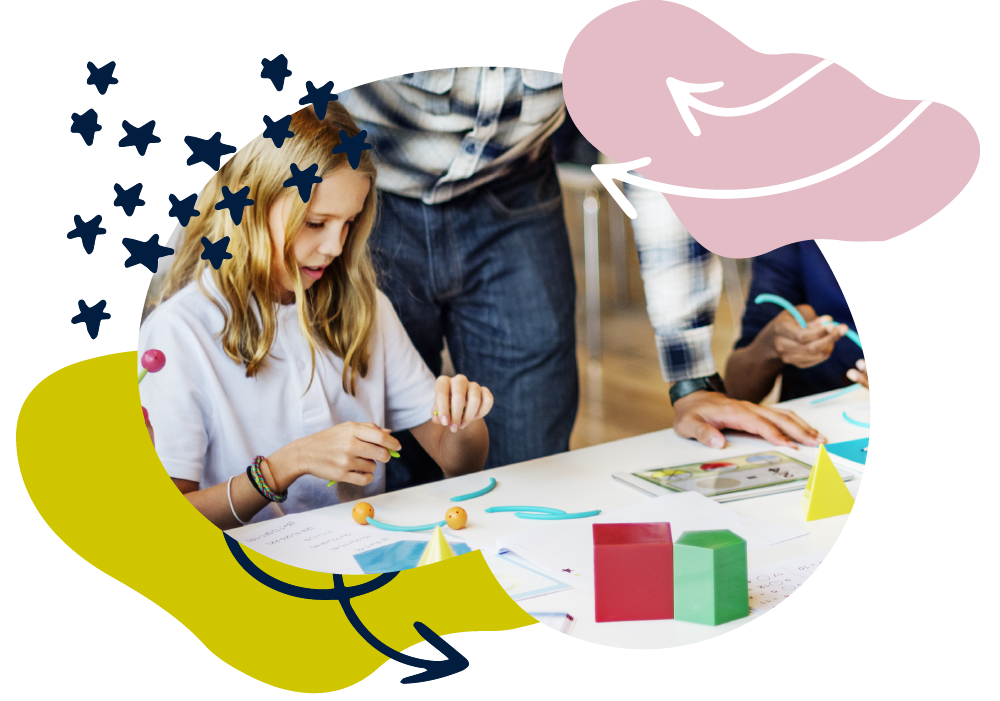We use cookies to enhance your experience on our website. By continuing to use our website, you are agreeing to our use of cookies. You can change your cookie settings at any time. Find out more# Maths: Age 10–11 (Year 6)

In Year 6, your child will apply the maths they already know alongside developing new skills to help solve more complex problems. They will work with numbers up to 10 million and begin to learn about algebra and ratio. They will convert measurements, calculate volumes and learn about circles. They will draw and interpret pie charts and find averages.

They’ll be taught long division for dividing four-digit by two-digit numbers. They’ll be able to use brackets in calculations and explain remainders. They’ll learn to multiply and divide with fractions and decimals, and calculate percentages.

Don’t worry if some methods that your child learns are new to you! There are still simple things you can do at home to support their development.

## How to help at home

You don’t need to be an expert to support your child with maths or help them develop a good sense of number! Here are three simple, but effective learning ideas that you can try with your child using everyday items at home.

### 1. Look for large numbers

Talk about large numbers in the real world, such as house prices, electricity meter readings or football transfer values. When you see big numbers, ask your child to read them aloud. Can they write large numbers accurately if you say them?

### 2. Rewrite the recipe book

Cooking is a great way to explore ratio and proportion. Try adjusting recipes to make different quantities. For example, ask your child: ‘A recipe uses 240 g of oats to make 18 flapjacks. What quantity of oats is needed for 24 flapjacks?’.

### 3. Find an average

Explore the ‘mean’ average in real life. Find a football team’s mean number of goals per match by adding all the goals scored and dividing the total by the number of matches played. Or find the mean number of minutes the school run takes over a week.

You’ll find more activity ideas in our Kids’ activities section

## What your child will learn at school

#### Number & place value in Year 6 (age 10–11)

In Year 6, children will be expected to read, write, order, compare and round numbers up to 10,000,000 and begin to learn about algebra, ratio and proportion. This includes:

• using number lines to add and subtract negative numbers
• using simple formulae and following rules such as 2n + 3 to find numbers in a sequence
• solving problems involving place value, ratio, scale factors, and equations expressed

#### Addition & subtraction in Year 6 (age 10–11)

In Year 6, children will be expected to be able to solve problems, including multi-step word problems, involving adding, taking away, multiplying and dividing with large numbers. This includes:

• choosing efficient methods to solve problems and checking their answer using a different method
• exploring the order of operations using brackets
• rounding answers to a specified degree of accuracy, for example, to the nearest 10, 20, 50, and so on.

#### Multiplication & division in Year 6 (age 10–11)

In Year 6, children will be expected to be able to multiply and divide with large numbers using formal written methods including long division. This includes:

• multiplying four-digit numbers by two-digit numbers using long multiplication
• dividing four-digit numbers by two-digit numbers using long division
• solving multi-step problems using addition, subtraction, multiplication and division choosing which methods to use and explaining why.

#### Fractions, decimals & percentages in Year 6 (age 10–11)

In Year 6, children will be expected to be able to calculate and solve problems using fractions, decimals and percentages. This includes:

• using common factors to simplify fractions
• multiplying pairs of fractions and dividing fractions by whole numbers
• multiplying one-digit numbers with up to two decimal places by whole numbers, for example 1.25 x 2.

#### Geometry in Year 6 (age 10–11)

In Year 6, children will be expected to be able to solve problems by calculating and converting measurements with up to 3 decimal places, including between miles and kilometres. This includes:

• working out areas of shapes including triangles and parallelograms
• working out volumes of cubes and cuboids
• knowing and using a formula to work out areas and volumes of some shapes.

#### Measurement in Year 6 (age 10–11)

In Year 6, children will be expected to be able to solve problems by calculating and converting measurements with up to 3 decimal places, including between miles and kilometres. This includes:

• working out areas of shapes including triangles and parallelograms
• working out volumes of cubes and cuboids
• knowing and using a formula to work out areas and volumes of some shapes.

#### Statistics in Year 6 (age 10–11)

In Year 6, children will interpret and present discrete and continuous data in a range of ways. This includes:

• connecting work on angles, circles, fractions, and percentages to make and interpret pie charts
• solving problems by interpreting and making line graphs
• calculating and interpreting mean averages.

#### Ratio and proportion in Year 6 (age 10–11)

In Year 6, your child will begin to learn about ratio and proportion. This includes:

• solving ratio problems using their knowledge of multiplication and division
• using their knowledge of percentages and the fact that the number of degrees in a circle is 360° to calculate angles of pie charts
• solving problems involving unequal sharing and grouping using their knowledge of fractions and multiples.